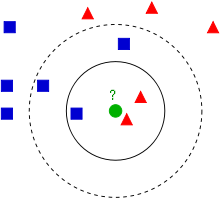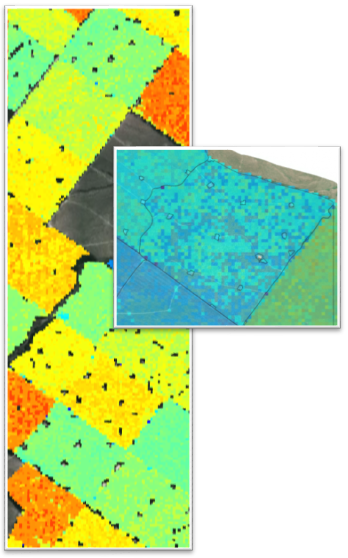+64 7 350 3209 +61 2 8011 3645 info@interpine.co.nz
Select Page

Over the last 3 years Interpine have been developing a range of LiDAR analysis techniques for the imputation of forest yield metrics of wide areas of forests estates. Interpine have focused its research in late 2012 to a statistical technique known as k-Nearest Neighbour (k-NN) imputation. k-NN is a type of instance-based learning (machine learning algorithms) and under a k-NN approach the forest parameters of a given patch of forest are assigned based on its similarity, in statistical terms, to a set of reference observations for which there are both LiDAR and ground measurements.

Figure 1 shows a simplistic example of k-NN classification. The test sample (green circle) should be classified either to the first class of blue squares or to the second class of red triangles. If k = 3 (solid line circle) it is assigned to the second class because there are 2 triangles and only 1 square inside the inner circle. If k = 5 (dashed line circle) it is assigned to the first class (3 squares vs. 2 triangles inside the outer circle). If k = 1 then the red triangle is the closest and the sample is assigned to the second class of red triangles.Figure 1 Simple classification concept diagram for the use k-NN;      Source: http://en.wikipedia.org/wiki/KNN

kNN imputation has the following properties that make it a favourable technique for resource assessment purposes:

• It offers favourable integration with the current yield prediction framework of the majority of New Zealand’s forest management companies;
• kNN has the ability to extrapolate a small number of reference plots to deliver precise information about a large number of stands;
• The technique is non-parametric and free from distributional assumptions.

Implementation with the Use of LiDAR Data.

A LiDAR data cloud can be characterised to produce hundreds of LiDAR metrics across the forest area of interest. Concurrently ground measurements can be obtained using bounded field plots, allowing the calculation of forest parameters such as total recoverable volume (TRV), and stocking and also log product volumes. At each ground plot a high grade survey GPS unit will be used to fix the plot centre to sub 0.5m accuracy and these plot centres were used to produce LiDAR metrics for the part of the LiDAR point cloud that was exactly concurrent with the ground plot. This results in the production of two datasets one which contained ground plot measurements and LiDAR metrics, referred to as the reference dataset, and the other containing LiDAR metrics only at a pixel resolution suitable for the forest type, which is referred to as the target dataset.

The kNN technique uses patterns in the LiDAR data to identify which plot in the reference dataset is most similar to each pixel in the target dataset. The most similar reference plot is known as the nearest neighbour and the number of neighbours used as donors is referred to as k. Under a scenario of k=1 the single nearest neighbour from the reference dataset provides all the response variables (e.g. TRV, stocking, product mix) which had been recorded during ground plot measurement. The LiDAR metrics in both the target and the reference dataset are used to define the proximity of neighbours and candidate predictor variables. It is important to select only the most important candidates while removing  unimportant ones and this can be done using algorithms based on a technique called simulated annealing. The selected variables were used to impute the desired response variables for every cell in the target dataset. In this manner the measurements recorded in the ground plots were extrapolated across the entire study area using the information derived from the LiDAR dataset.Figure 2. Raster of the target dataset with pixels resulting from a response variable for an area of interest.

We would like to acknowledge the work is conducted with Future Forest Research, with detailed written science papers available to Future Forest Research Members.